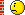# Superimpose TGraphErrors graph and TF1 function

I want to superimpose a TGraphErrors graph and a TF1 function (the fitting function). I tried with

``````TCanvas *c1 = new TCanvas("c1", "Fit", 700, 500);

TGraphErrors *gr1 = new TGraphErrors(n,x,y,ex,ey);
gr1->Draw("AP");

TF1 *func = new TF1("func", "*exp(x/)+", 0, 12);
func->SetParameters(0.2,98,0);
func->Draw("same");``````

but I can see only the graph’s data.
Can someone help me?
Thank’s

Actually it should work this way.

Try this code:

[code]void test(){
const Int_t n=20;
Double_t x[n],y[n],ex[n],ey[n];
for (Int_t i=0; i < n; i++) {
x[i]=i0.1;
y[i]=10
sin(x[i]+0.2);
}
for (Int_t i=0;i<n;i++){
ex[i]=0.05;
ey[i]=0.1;
}
TCanvas *c=new TCanvas(“c”,“name”,600,600);
TGraphErrors gr1 = new TGraphErrors (n,x,y,ex,ey);
gr1->Draw("AC
");

TF1 *func=new TF1(“func”,"*x+",0,2);
func->SetParameters(3,0.5);
func->Draw(“same”);
} [/code]

I can see both, TGraphErrors and the TF1 function…

Cheers

I tried your code. It works!
But my script doesn’t.I attach it so you can verify if there are errors.
graph.C (554 Bytes)

Sorry, I can’t find any errors…

It’s strange, the only difference between the two scripts seems to be that you didn’t fill the array using loop…

simply change

``` TF1 *func = new TF1("func", "*exp(x/)+", 0, 12); ```to

``` TF1 *func = new TF1("func", "*exp(x/)+", 0, 400); ```
Rene